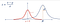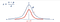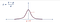# Standard Normal Distribution and z-score/Z-Statistic

Any distribution can be standardized. Assume the population mean is µ and standard deviation is σ , a distribution is in it’s standard form when (µ,σ2) = (0,1).

Normal distribution can also be standardized using the formula

# z = (x — µ)/σ

Where z is the Z score. Consider the below normally distributed data points.Normal distribution depicted by blue curve

When every data point x is subtracted from the accurate representation of population mean i.e sample mean, we move the graph towards the origin and the µ becomes zero as shown below.For (x — µ) sample, µ = 0

After subtracting each data point by sample mean, the new sample mean becomes zero and the graph is moved with origin becoming the central axis. The standard deviation does not change due to this and the shape of the graph remains the same. Now we divide the data points of the above obtained distribution by σ, we now obtain the below distribution.When (x — µ) is divided by σ, σ =1

Our new standard deviation of the sample becomes one and there is a change in shape of the graph, it flattens out. The new distribution is called the standard normal distribution and is denoted by Z~(0,1) where ~ is the distribution and 0 and 1 are mean and standard deviation respectively.

## Why Standardization?

In addition to normalization of the distribution, standardization further makes inferential statistics easier to calculate and also comparing two standard normal distributions is much accurate.

Hi good to see y'all, I am an aspiring data analyst and will be posting stuff about Statistics, Python and R and also some interesting projects I do. B-)

Hi good to see y'all, I am an aspiring data analyst and will be posting stuff about Statistics, Python and R and also some interesting projects I do. B-)